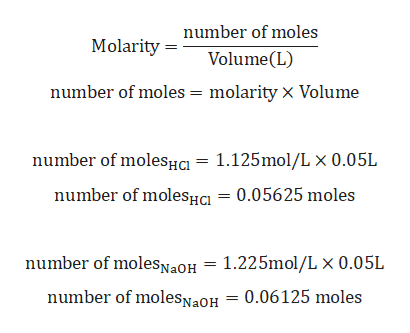The reaction of hydrochloric acid and sodium hydroxide is a very rapid and exothermic reaction.The equation is: HCl(aq) + NaOH(aq)→NaCl(aq) + H2O(l) In one experiment, a student placed 50.0 mL of 1.125 M HCl at 25.5º C (density = 1.02 g/mL) in acoffee cup calorimeter. To this, the student added 50.0 mL of 1.225 M NaOH also at 25.5º C (density = 1.04 g/mL). The mixture was stirred, and the temperature quickly increased to a maximum of 32.2º C. a. Determine the limiting reactant.b. Calculate the mass of the solution once the HCl (aq) and NaOH (aq) are mixed.

Question
The reaction of hydrochloric acid and sodium hydroxide is a very rapid and exothermic reaction.
The equation is: HCl(aq) + NaOH(aq)→NaCl(aq) + H2O(l)

In one experiment, a student placed 50.0 mL of 1.125 M HCl at 25.5º C (density = 1.02 g/mL) in acoffee cup calorimeter. To this, the student added 50.0 mL of 1.225 M NaOH also at 25.5º C (density = 1.04 g/mL). The mixture was stirred, and the temperature quickly increased to a maximum of 32.2º C.

a. Determine the limiting reactant.
b. Calculate the mass of the solution once the HCl (aq) and NaOH (aq) are mixed.
Step 1

Given:

Molarity of HCl (MHCl) = 1.125 M.

Volume of HCl (VHCl) = 50.0 mL= 0.05 L.

Molarity of NaOH (MNaOH) = 1.225 M

Volume of NaOH (VNaOH) = 50.0 mL= 0.05 L.

Balanced chemical reaction is given by:

HCl(aq) + NaOH(aq)→NaCl(aq) + H2O(l)

Step 2

Calculation for Number of moles of HCl and NaOH:help_outlineImage Transcriptionclosenumber of moles Molarity Volume (L) number of moles = molarity X Volume number of molesHc 1.125mo1/L x 0.05L number of molesHa = 0.05625 moles number of molesNaOH = 1.225mol/L x 0.05L number of molesNaOH 0.06125 moles fullscreen
Step 3

Calculation for mass of HCl and NaOH:

Density of HCl solution = 1...

Want to see the full answer?

See Solution

Want to see this answer and more?

Our solutions are written by experts, many with advanced degrees, and available 24/7

See Solution
Tagged in

Solutions